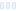# Simply Maize

## Cold cereal - Kashi Company

Main info:

Simply Maize
Cold cereal - Kashi Company
3/4 cup
100.0 Calories
23.0 g
1.0 g
2.0 g

2.0 g
0 mg
0 g
110.0 mg
0 g
0 g

Percent calories from...
Nutrition Facts
For a Serving Size of (g)
How many calories are in Simply Maize? Amount of calories in Simply Maize: Calories Calories from Fat (%)
% Daily Value *
How much fat is in Simply Maize? Amount of fat in Simply Maize: Total Fat
How much sodium is in Simply Maize? Amount of sodium in Simply Maize: Sodium
How much potassium is in Simply Maize? Amount of potassium in Simply Maize: Potassium
How many carbs are in Simply Maize? Amount of carbs in Simply Maize: Carbohydrates
How many net carbs are in Simply Maize? Amount of net carbs in Simply Maize: Net carbs
How much fiber is in Simply Maize? Amount of fiber in Simply Maize: Fiber
How much glucose is in Simply Maize? Amount of glucose in Simply Maize: Glucose
How much protein is in Simply Maize? Amount of protein in Simply Maize: Protein
Vitamins and minerals
How much Vitamin A is in Simply Maize? Amount of Vitamin A in Simply Maize: Vitamin A
How much Vitamin C is in Simply Maize? Amount of Vitamin C in Simply Maize: Vitamin C
How much Calcium is in Simply Maize? Amount of Calcium in Simply Maize: Calcium
How much Iron is in Simply Maize? Amount of Iron in Simply Maize: Iron
Fatty acids
Amino acids
* The Percent Daily Values are based on a 2,000 calorie diet, so your values may change depending on your calorie needs.Loading similar foods...
Note: Any items purchased after clicking our Amazon buttons will give us a little referral bonus. If you do click them, thank you!Be cool

- Zen orangeI never skip arm day

- Buff broccoli